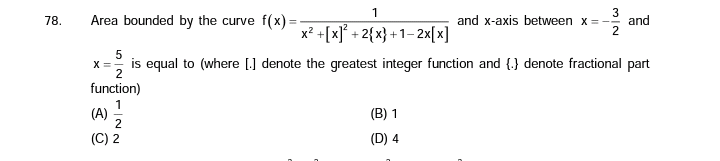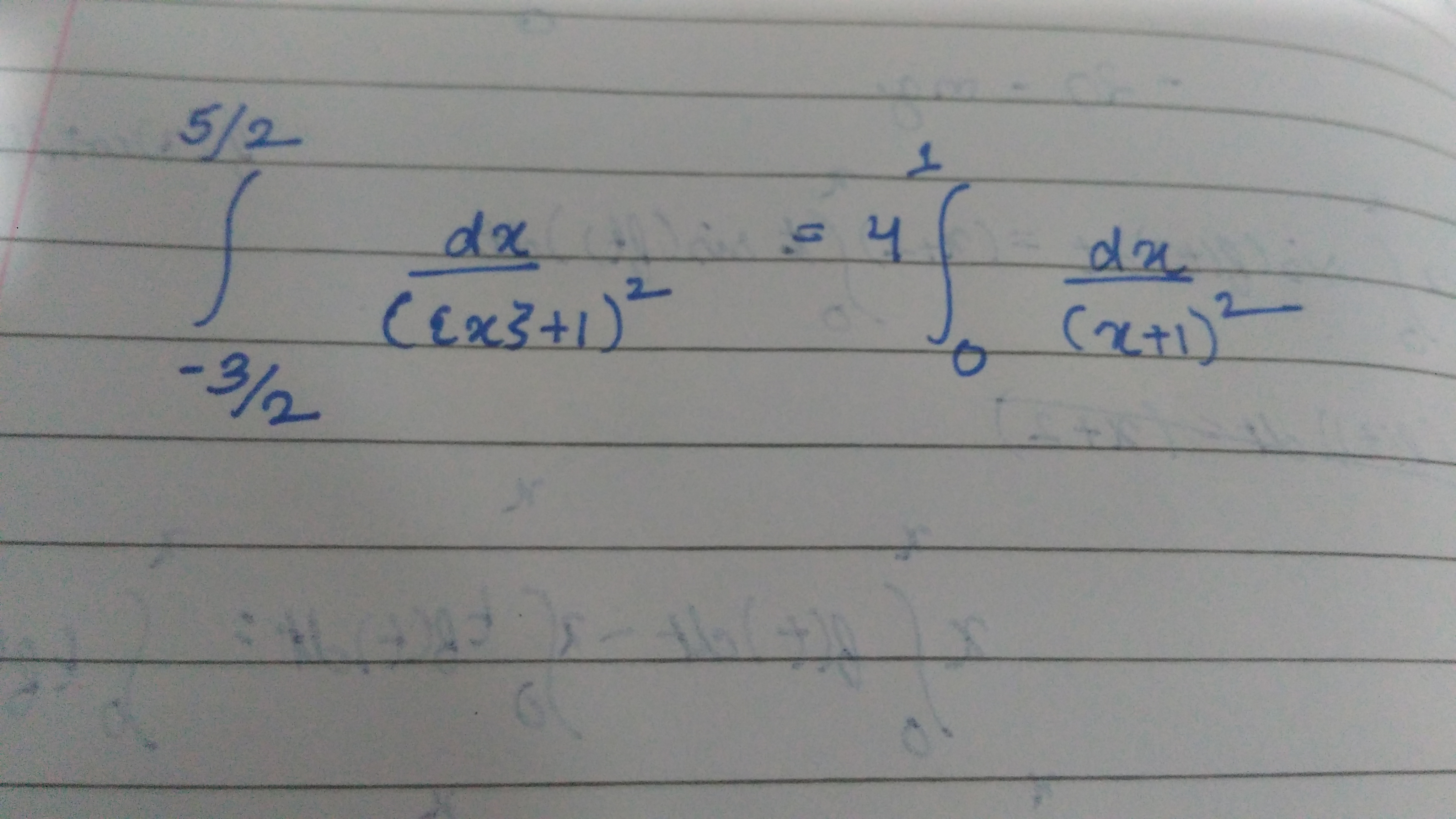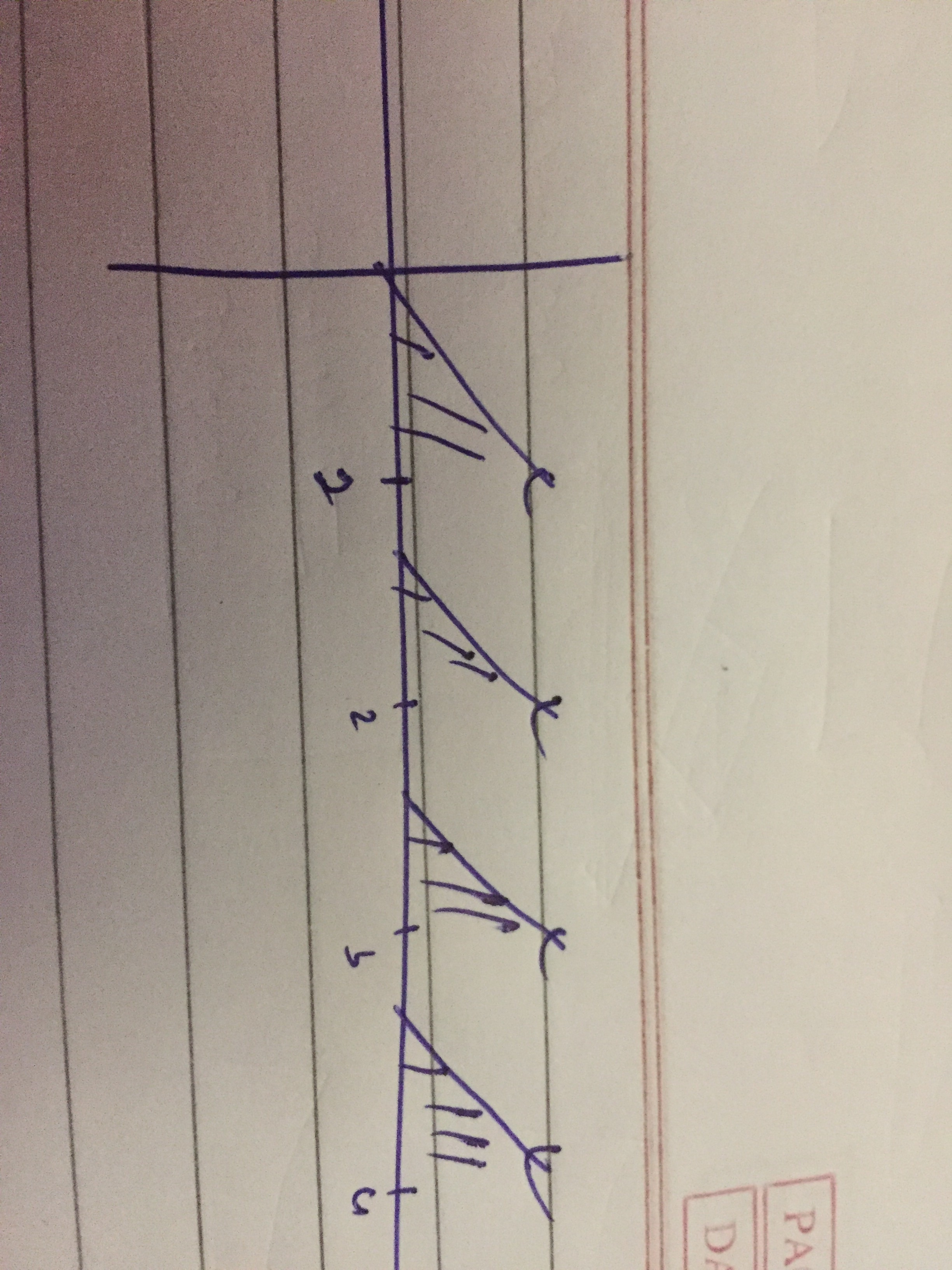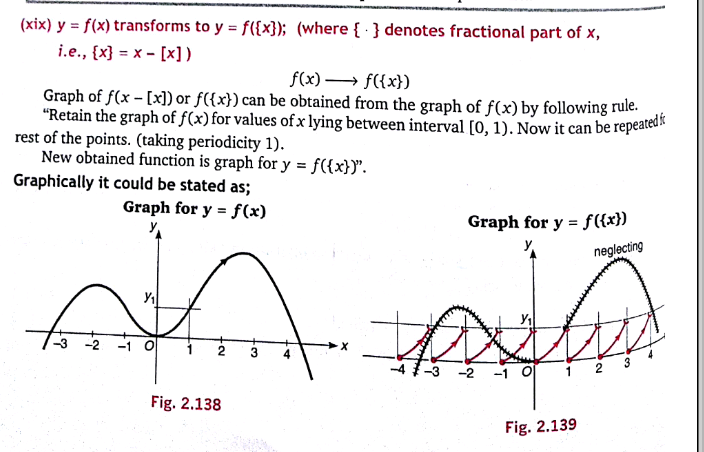# Doubt from Area under curveHow do I draw graph of 1/({x}+1)^2 ?pls check if this is correct

Whenever you need to integrate fractional part type equation then you have to break the integration about integeral values and then integrate
For example for integrating {x} from 0 to 1.7
Then in interval 0 to1 it is x
In interval 1 to 1.7it is x-1
So integrate x from 0 to 1 and add the integration of x-1 from 1 to 1.7 in it

How did you break the limits into 0 to 1 ?

@Raghav_2020 because area covered by x from 0 to 1 is same as area covered by x-1 from 1 to 2 and so on
Basically area covered by {x} from 0 to 4 = 4 area covered by {x} from 0 to 1With similar reasoning you’ll get the above result

Ok. ThanksPls read this books...functions_and_graphs_gelfand.pdf (2.8 MB)